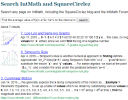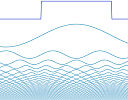Search IntMath
Close

450+ Math Lessons written by Math Professors and Teachers

5 Million+ Students Helped Each Year

1200+ Articles Written by Math Educators and Enthusiasts

Simplifying and Teaching Math for Over 23 Years

# IntMath Newsletter: Search math, Fourier, DeepMind

By Murray Bourne, 25 Apr 2019

25 Apr 2019

1. New on IntMath: How to search math
2. Resources: Fourier, breaks
3. Math in the news: DeepMind, bees
4. Math movies: vectors, collection
5. Math puzzle: Sequence
6. Final thought: You can't eat coal

## 1. New on IntMath: How to search math

I recently updated the search function on IntMath, so users would have an ad-free experience and not be taken to unrelated sites.

It's been interesting observing the searches that readers have been performing (I have no idea who you are, of course - I just have a record of what has been searched for.)

While everyone assumes students are "tech wizards" and are have highly developed search skills, many of the searches being made have no hope of success. I felt it was time for an advice article.A lot of students struggle to find answers for their math questions. Here are some helpful hints. How to search for solutions to math questions

## 2. Resources

### (a) Interactive Introduction to Fourier Transforms

This one goes into the category of something I wish I had the time to do myself!

Fourier transforms are a neat way of analyzing and solving engineering and science problems, especially those involving electronics.Don't miss Jez Swanson's brilliant exploration, no matter your level of math knowledge:

Fourier Series (on IntMath) is a branch of Fourier analysis, especially useful for periodic functions (ones that repeat a pattern at regular intervals).

### (b) Swot or rest for best learning?

The brain needs tiome to process and absorb new information - that's a main function of sleep, of course.

This new research backs up what many of us already know - taking breaks is essential for effective learning.This research refers to learning a physical skill, but it's not hard to imagine they'll find similar results when they extend it to other types of learning. See:

## 3. Math in the news:

### (a) DeepMind nearly flunks high school math

Google's DeepMind is the "world leader in artificial intelligence research and its application for positive impact." [source]

However, recently the DeepMind team tried to teach their artificial intelligence neural networks some basic math, and it didn't fare so well. The topics were basic problems in arithmetic, algebra and calculus, and DeepMind only achieved 14 out of 40 questions on a standard test.

It did best on tasks like determinining the place value (e.g. "Which digit is in the hundreds place in the number 23,741?") and it's worst performance was for converting a number from one base to another (e.g. "What is 4351 base 8 in base 10?")

### (b) However, honey bees can do (simple) math

An interesting surprise was this research, which showed honeybees can not only count, but can do some very simple addition and subtraction, and decide "greater than" or "less than".

## 4. Math Movies

### (a) What is a vector?This is a brief, painless introduction to the important concept of vectors, by David Huynh.

### (b) Charting cultureThis is an interesting data visualization which tracks how culture and scientific ideas spread around the world.

## 5. Math puzzles

The puzzle in the last IntMath Newsletter asked about 4-digit numbers that give repeated digits when multiplied. A correct answer with sufficient reasons was submitted by Thomas, who actually submitted two different approaches. Special mention to Danesh and Tomas, who got very close.

### New math puzzle: Sequence

We have a sequence 3, −2, 3, −2, 3, −2,.... Find a formula for the n-th term.

You can leave your response here.

## 6. Final thought - You can't eat coal

There's a fierce debate going on as Australian politicians are in full campaign mode for the federal election next month.

One of the hot issues is a new coal mine set for development in Queensland state, home of the Great Barrier Reef and for the most part, has a very dry climate.

There was a last-minute approval for the mine at federal level, but it's yet to receive the go-ahead from the state government. In what is shaping up as the "climate election", here's some analysis from The Guardian:

As alternative energy sources become cheaper, it seems fundamentally short-sighted (and extraordinarily stupid) to ruin farmland and underground water for coal dollars.

The company bidding for the rights to operate the mine is Adani, an India-based company. India is already home to 9 of the top 10 polluted cities in the world - and they want to make them even dirtier?

Until next time, enjoy whatever you learn.

1. Gayathri Chakravarthy says:

The nth term is 0.5 + 2.5(-1)^(n-1)

2. Christopher Odoom says:

The sequence 3,-2,3,-2,...
Has the nth term as :
An =(-5/2 +1/2 (-1)^n)*cos(n*pi)+5*cos(n*pi)
Where n = 0,1,2,3,4,5,...

3. james.v says:

let a(0)= 3; a(1)= -2
Then i would like to construct a recurrence relation
a(n) = a(n-2)^2-a(n-1)^2 +a(n-1).
It generate this sequence.

4. Larry Dreaves says:

Nth term = 0.5 - 2.5 x (-1)^n

5. Russell Wild says:

The alternate positive and negative signs are generated by including the factor (-1)^n.
Now all
All we have to do now is generate is 3,2,3,2,....
Notice that these terms can be written 2 1/2 + 1/2, 2 1/2 - 1/2, 2 1/2 + 1/2, ....
Notice that 2 1/2 occurs in every term.
So we can write 2 1/2 + (-1)^n x 1/2
Thus, all together, we have Tn= (-1)^n[2 1/2 - (-1)^n x 1/2]
We can simplify this expression to
Tn= -1/2[5(-1)^n - (-1)^2n]
Notice that the portion of this expression (-1)^2n is always equal to 1, thus we can simplify the expression to
Tn= -1/2[5(-1)^n - 1]
A little more simplification brings the expression to:

Tn = 1/2[1 - 5(-1)^n]

6. Arun kumar says:

{xn} where xn = 3 if n odd
-2 if n even

7. Arun Kumar A says:

Nth term is (1/2)-(5/2)(-1)^n

8. Michael says:

3,-2,3,-2

Can be the sum of
2,-2,2,-2,...
+
1,0,1,0,...

Which can be expressed as (for n>=0)
2*(-1)^(n)
+
((n+1)%2)

9. Nicola Marinelli says:

n integer, >0
X0=-2
(((-1)^(n-1))*5)+Xn-1

### Comment Preview

HTML: You can use simple tags like <b>, <a href="...">, etc.

To enter math, you can can either:

1. Use simple calculator-like input in the following format (surround your math in backticks, or qq on tablet or phone):
a^2 = sqrt(b^2 + c^2)
(See more on ASCIIMath syntax); or
2. Use simple LaTeX in the following format. Surround your math with $$ and $$.
$$\int g dx = \sqrt{\frac{a}{b}}$$
(This is standard simple LaTeX.)

NOTE: You can mix both types of math entry in your comment.